GCSELevel 4-5Cambridge iGCSEWJEC

Quadratic graphs can be sketched on a set of axes, and from here the roots can be found.

Careful: In some cases, the equation may need to be rearranged to the form of a quadratic.

## Sketching Quadratic Graphs and Finding the Roots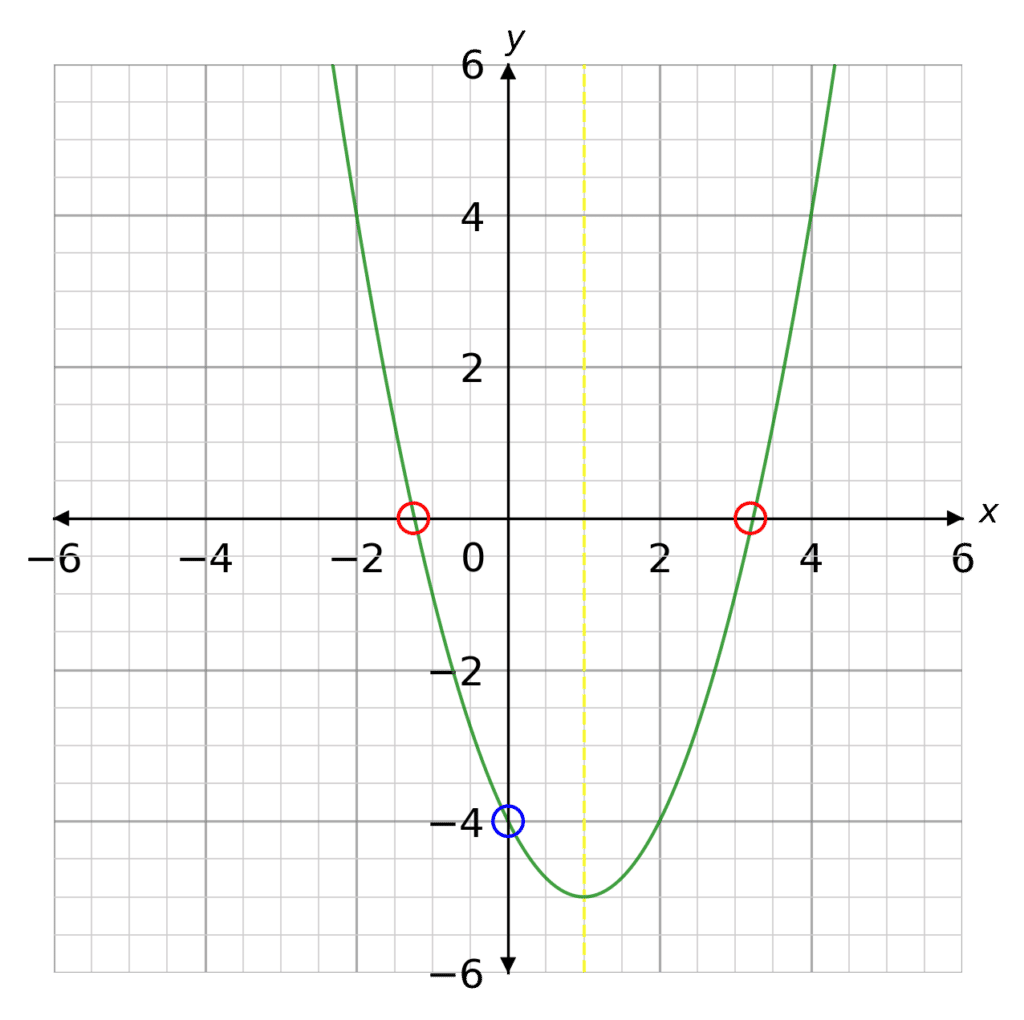$y=x^2+bx+c$

They have a general U shape, with one line of symmetry halfway between the $x$ intercepts. The $x$ intercepts are circled in red. The $y$ intercept is circled in blue.

Sketching:

To sketch a quadratic you can create an $xy$ table, for a selection of $x$ values, and plot the coordinates. Connecting these points will create the shape of your quadratic.

Roots:

To find the roots of the quadratic equation, you take a look at your sketch and see where the graph crosses the $x$ axis.

Note: The roots of a quadratic equation are the same as the $x$ intercepts.

Take a look at the examples below.

Level 4-5GCSEWJECCambridge iGCSE

## Example 1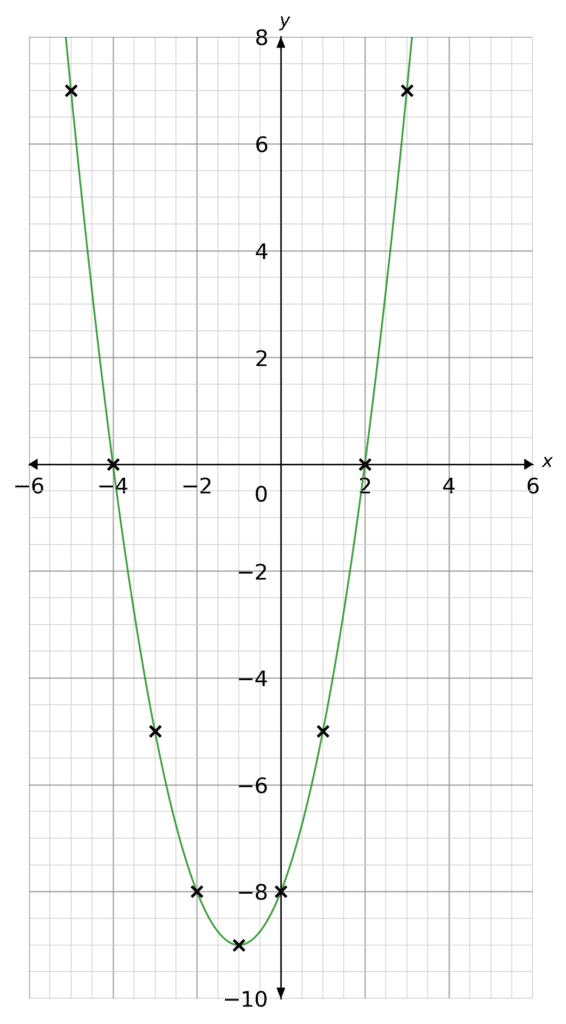Plot the following graph on a set of $x$ and $y$ axes,

$y=x^2+2x-8$

Hence, find the roots of the equation.

[3 marks]

Creating The $xy$ Table

Substituting the values $x=-5$ to $x=3$, we get the following table: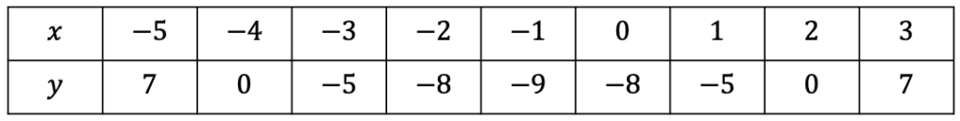Plotting these points as coordinates we get the following graph (as seen on the right).

Finding the Roots

From the sketch we can see the graph crosses the $x$ axis at $-4$ and $2$. These are the roots.

Level 4-5GCSEWJECCambridge iGCSE

## Example 2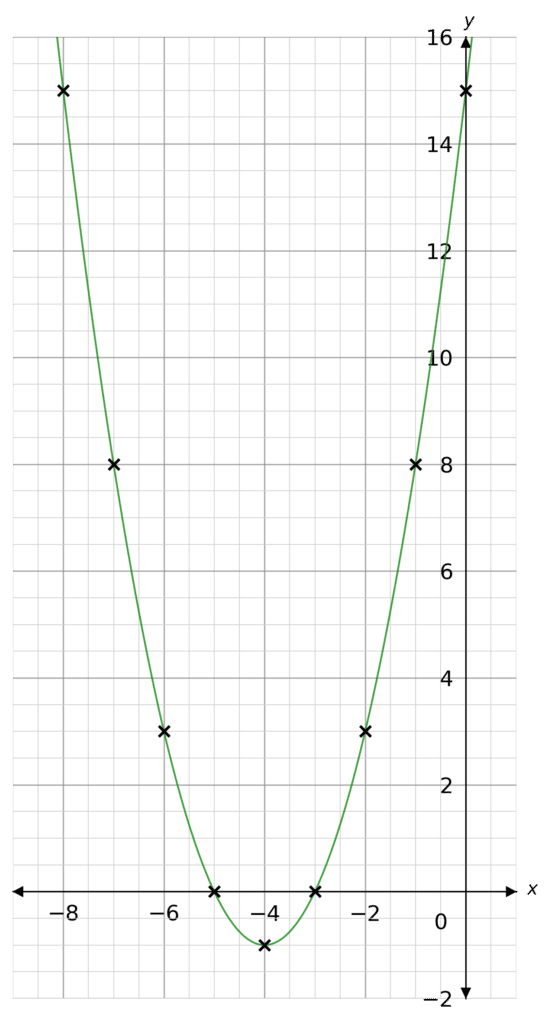Plot the following graph on a set of $x$ and $y$ axes,

$y=x^2+8x+15$

Hence, find the roots of the equation.

[3 marks]

Creating The $xy$ Table

Substituting the values $x=-8$ to $x=0$, we get the following table: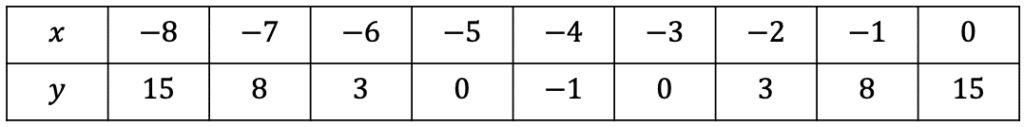Plotting these points as coordinates we get the following graph (as seen on the right)

Finding the Roots

From the sketch we can see the graph crosses the $x$ axis at $-5$ and $-3$. These are the roots.

Level 4-5GCSEWJECCambridge iGCSE

$y=x^2+7x+10$

Creating The $xy$ Table

Substituting the values $x=-7$ to $x=0$, we get the following table: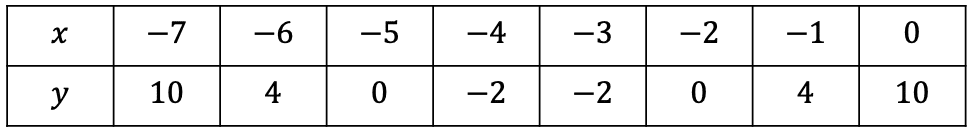Plotting these points as coordinates we get the following graph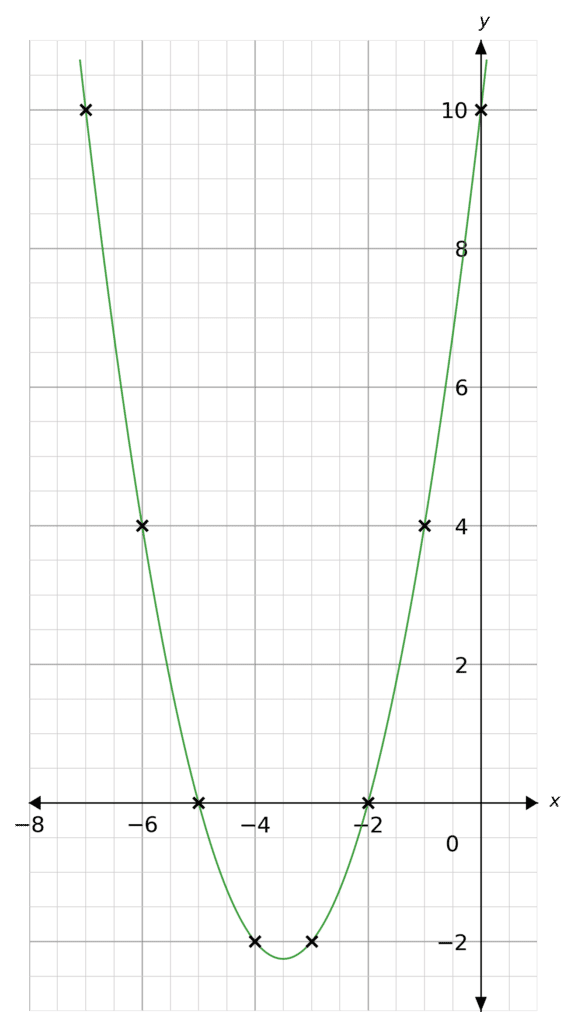Finding the Roots

From the sketch we can see the graph crosses the $x$ axis at $-5$ and $-2$. These are the roots.

$y=x^2-9x+14$

Creating The $xy$ Table

Substituting the values $x=0$ to $x=9$, we get the following table: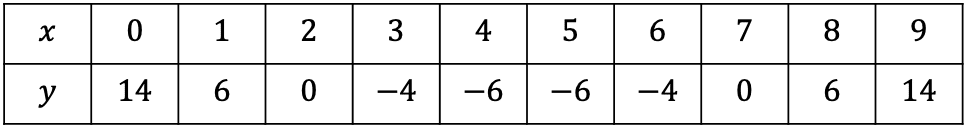Plotting these points as coordinates we get the following graphFinding the Roots

From the sketch we can see the graph crosses the $x$ axis at $2$ and $7$. These are the roots.

This question we first need to rearrange the equation to the form of a quadratic:

$y=x^2+2x+1$

Creating The $xy$ Table

Substituting the values $x=-8$ to $x=0$, we get the following table: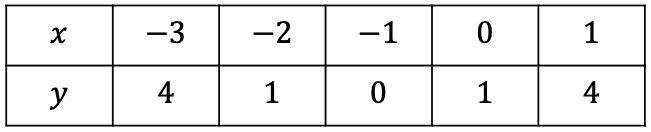Plotting these points as coordinates we get the following graph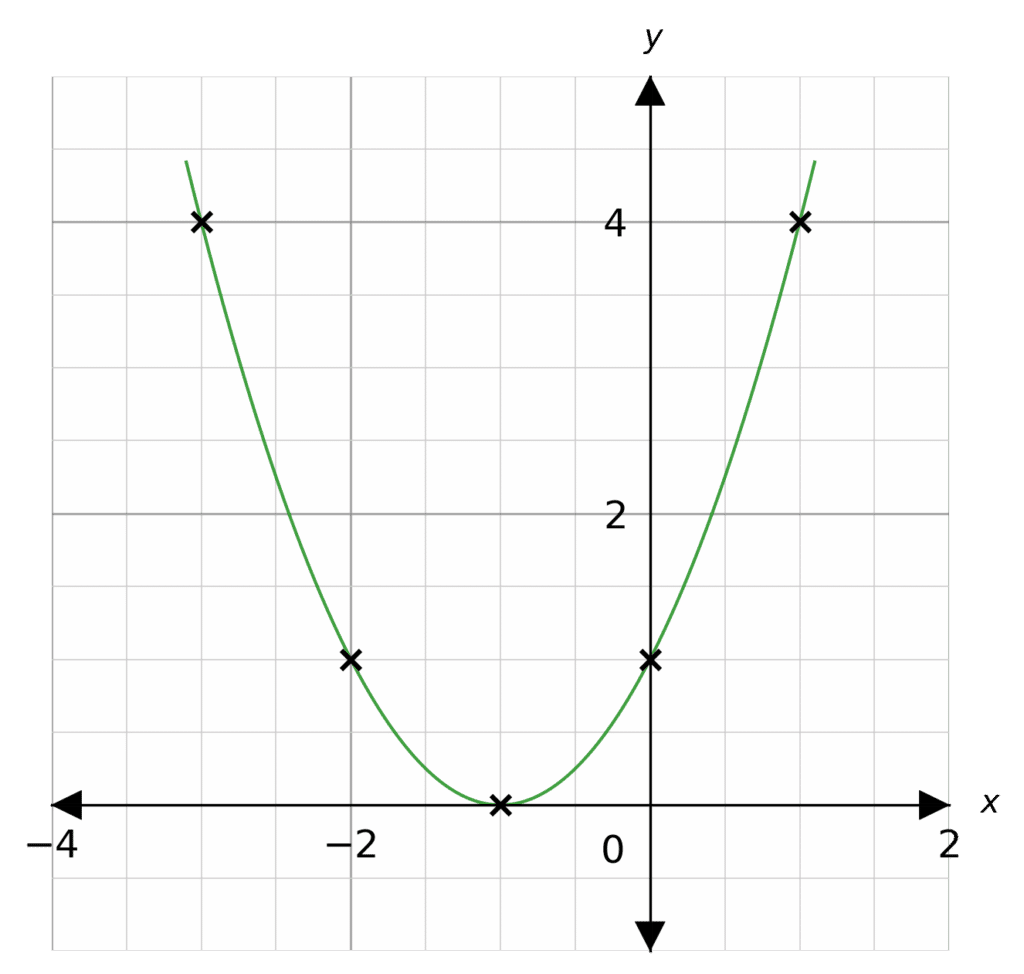Finding the Roots

From the sketch we can see the graph touches the $x$ axis at $-1$. For this particular question the quadratic equation only has one root ($-1$

## You May Also Like...### MME Learning Portal

Online exams, practice questions and revision videos for every GCSE level 9-1 topic! No fees, no trial period, just totally free access to the UK’s best GCSE maths revision platform.

£0.00